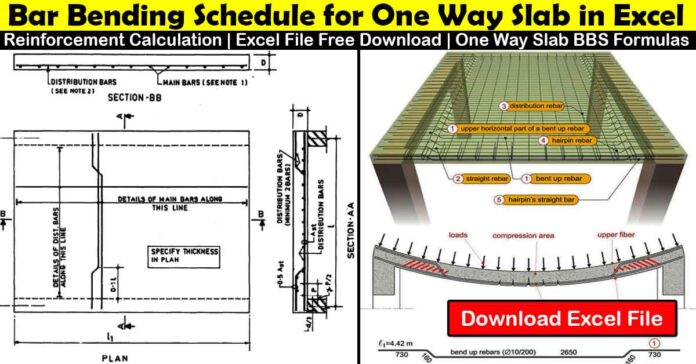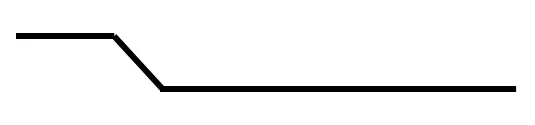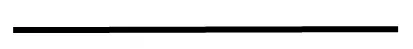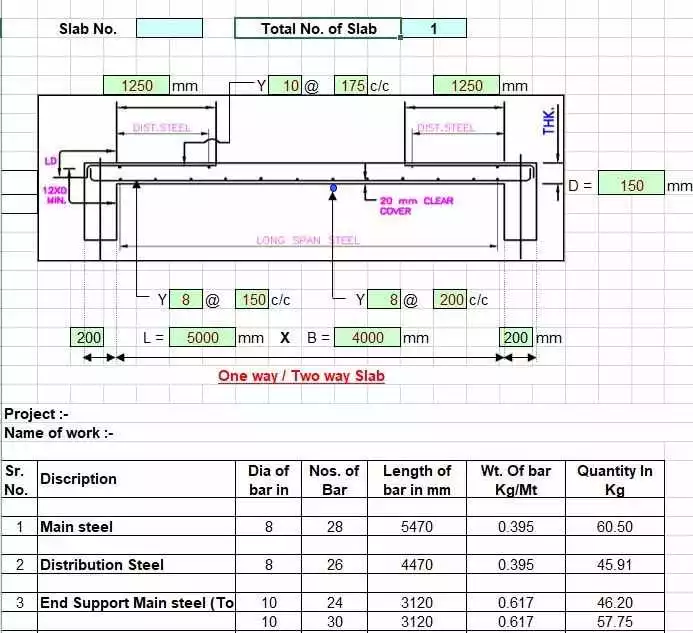# Calculate Bar Bending Schedule for One Way Slab | Steel Quantity for One Way Slab

Bar bending schedules are vital in determining the quantities of reinforcement used in steel or concrete slabs. To find out bar bending schedules of slab, you should learn first Bar Bending Schedule Formula or Basics of Bar Bending Schedule and how to use concrete covers. We will also discuss about the rebar for concrete slab calculator, calculation formula, calculate rebar spacing, slab of steel quantity, reinforcement bbs bar bending schedule for slab and many more about the steel quantity for one way slab

## Bar Bending Schedule for Slab:

In construction, there are 16 different slab varieties. Well, the thickness of a slab typically ranges from 4 to 8 inches. Generally, our slabs are 6′′ (0.15m) thick. We use slabs that are 8′′ and over in thickness for occasional heavy loads.

Before calculating the BBS of One Way Slab, we should know about the function and detail of one way slab;

### One Way Slab:

One way slabs are those in which the ratio of longer spans (L) to shorter spans (B) is equal to or greater than 2. In a one-way slab, the load is carried in one direction, where main bars are designed, and in the opposite direction, where thin bars—known as distribution bars—are used instead of main bars to distribute the load.

One way slab = Longer span / shorter span > 2

## Reinforcement Required for Steel Quantity of One Way Slab & Two Way Slab:

Generally, slabs are classified into two types One-way Slab and Two-way slab, In one-way slab, Main bars run in the shorter direction (called Cranked bars) and distribution bars run in the longer direction (called Straight bars). In two-way slabs, Main bars are available in both directions.

## Bars used in One Way Slab:

### Distribution bars:

These bars are straight bars

### Main bars:

These bars have a crank. The main bars have a length of 0.42D and are cranked at a 45-degree angle.

Where, D = Depth of Slab- Top cover – Bottom cover

### Extra Bars:

To maintain the slab’s structural stability, an additional bar is provided at the bottom of the Cranked bars.

L/4 is the length of the Extra bar ….. (rebar calculation formula)

### Steps to calculate the Reinforcement required for Slab:

1. Subtract the slab clear cover from the distribution and main bar length calculations.
2. Determine the value of “D” (Depth of slab- Top cover- Bottom cover)
3. Calculate the number of bars by using rebar calculation formula
4. Calculate the overall steel weight needed for slab reinforcement.

## Bar Bending Schedule for One Way Slab:

How to Calculate Rebar for Slab

### One way slab Reinforcement Detail:

Given Data:

1. Cranked bars or Main bar of diameter 16 mm and C/C spacing 150 mm.
2. Distribution bars of diameter 12 mm and C/C spacing 150 mm.
3. Top and bottom slab clear cover is 25 mm.
4. Side clear cover of RCC slab is 25 mm
5. Thickness of RCC slab is 150 mm.
6. Length of RCC slab is 6000 mm (longest side along beam)
7. Width of slab (Clear Span) without beam is 3000 mm. (shortest side which is perpendicular to beam)
8. Length of top extra bars = L/4 = 3000/4 = 750 mm
9. No. of top extra bars = No. of Main bars
10. Diameter of top extra bars

Now,

### For Main bars:Step 1:- Calculate numbers of main rods.

No. of Main Bars = (Length of Slab)/ Spacing + 1

No. of Main Bars = (6000)/150 + 1

No. of Main Bars = 40 + 1

No. of Main Bars = 41 Nos.

Step 2:- Calculate cutting length of one main rods (rebar of slab).

Cutting length of one crank bar = Clear span of slab + ( 2 x Development Length) + (1 x inclined length) – (2 x 45 Bend)

Cutting length of crank bars = 3000 + ( 2 x 40d) + (1 x 0.42D) – (2 x 1d)

(Development length = 40d, inclined length = 0.42D, 45 Bend= 1d)

Where,

D = Thickness of slab – 2 x Side clear cover – diameter of bars

D = 150 – 2 x 25 – 16 = 84 mm

D = 84 mm

Cutting length of one crank bars = 3000 + ( 2 x 40 x 16) + (1 x 0.42 x 84) – (2 x 16)

Cutting length of one crank bars = 3000 + 1280 + 35.28 + 32

Cutting length of one crank bars = 4347.28 mm

Step 3:- Calculate Total length of main rods.

Therefore Total length of crank bars = Length of one crank bar  x  No. of crank bars …. (steel calculation formula)

Total length of crank bars = 4347.28 x 41 = 178238.48 mm

Total length of crank bars = 178.238 meter.

Step 4:- Calculate total weight of main rods (rebar for slab).

W1 = (d2 / 162.25) x Length ….. (steel calculation formula)

W1 = (162 / 162.25) x 178.238

W1 = 281.23 Kg

### For Distribution bars:

To find the Distribution Bars Cutting Length we will follow the following steps:Step 1: – Calculate numbers of distribution rods.

To find the Bar Bending Schedule for Slab we use the following formula,

No. of Main bars = (Width of Slab/Spacing) + 1 …. (Rebar Calculation Formula)

No. of Main bars = (3000)/150 + 1

No. of Main bars = 20 + 1 = 21 Nos.

Step 2:– Calculate cutting length of one distribution bar.

Cutting length of one distribution bar = Length of Slab + 2 x development length

Cutting length of one distribution bar = 6000 + 2 x 40d

Cutting length of one distribution bar = 6000 + (2 x 40 x 12)

Cutting length of one distribution bar = 6000 + 960

Cutting length of one distribution bar = 6960 meter

Step 3:- Calculate Total length of distribution rods.

Therefore Total length of crank bars = Length of one crank bar  x  No. of crank bars

Total length of crank bars = 6960 x 21 = 146160 mm = 146.160 meter.

Step 4:- Calculate total weight of distribution rods.

W2 = (d2 / 162.25) x Length

W2 = (122 / 162.25) x 146.160

W2 = 129.72 kg

### For Top Extra bars:Length of top extra bar = L/4 = 3000/4 = 750 mm

No. of top extra bars = No. of Main bars = 41

Total length of Top extra bars = 750 x 41 = 30750 mm

Total length of Top extra bars = 30.750 meter

Therefore total weight of top extra bars = W3

W3 = (162 / 162.25) x 30.750

W3 = 48.52 Kg

### Total Weight of Reinforcement:

Hence,

Total Weight of Steel Rod = Total Weight of crank bars + Total Weight of Distribution bars + Total weight of extra top bars

Total Weight of Steel Rod = W = W1 + W2 + W3

Total Weight of Steel Rod = W = 281.23 kg + 129.72 kg + 48.52 kg

Total Weight of Steel Rod = W = 459.47 kg

Hence, the final calculate weight of steel quantity is 459.47 Kg

## Rebar Calculator for Slab:I sincerely hope you find this article on Bar Bending Schedule for One Way Slab to be useful and how to calculate steel quantity for One Way Slab.

### OTHER POSTS:

1. Basics of Bar Bending Schedule Formulas | BBS Formulas with Example

## What is the Difference between One Way Slab and Two Way Slab?

A one-way slab carries the load/weight perpendicular to the supporting beam in one direction. The load is carried in both directions in a two-way slab.

## What is D2/162 and D2/533 | Steel calculation?

Take into consideration that W = D2/162 kg/m is utilized when the bar length is specified in m. W = D2/533 kg/ft is utilized when the bar length is specified in ft.

## What is the formula of one way slab?

Greater span/smaller span equals Lx/Ly = 4500/2000 = 2.5, which is greater than 2. A one-way slab, then. In this slab, the distribution bars are on the longer side while the major reinforcement bars are on the shorter side.

## How do you calculate slab distribution bar?

Form = (total length with transparent cover) center to center spacing = 1 bar + main bar. The distribution bars of the metric are 125 + 1 + 2 = 2000 divided by 2 + 2 = 125 + 2 = 18 bars.

## What size concrete slab need rebar?

It can be used on slabs up to 5” thick, but it cannot fit on thinner ply sheets.

## What is the maximum spacing for rebar?

How do bars spread out on slabs according to IS-Code & IF Code? The spacing between the parallel main reinforced bars for RCC is 300 mm (3D). The minimum spacing between two primary parallel cables must be 450 mm or 5 D for this slab.

## How do you calculate rebar for footings?

Ensure the amount of rebar is required to support the foot. Generally, an 8 inch stick is sufficient. If your footings are 16 inches long, you will have to add three sticks on each side of your footing.

1.Monu Kumar
2.Shubhash